# NLP practice | BERT text classification and its magic modification (with Python code)

This article mainly introduces two text classification models: the BERT text classification basic model, and the magic modified model based on Bert and TextCNN. In the author’s actual work on text classification, the F1 value exceeded the Bert basic model by nearly 4%.

#### Technical exchange

Technology must be shared and communicated, and it is not recommended to work behind closed doors. One person can go very fast, and a group of people can go further.

Relevant information, data, and technical exchange improvements can be obtained by joining our communication group. The group has more than 2,000 members. The best way to make a note when adding is: source + direction of interest to facilitate finding like-minded friends.

Method ①, add WeChat ID: dkl88194, remarks: from CSDN + technical exchange
Method ②, WeChat search public account: Python learning and data mining, background reply: join the group

## 1. Baseline: Bert text classifier

The Bert model is a language model released by Google in October 2018. Once it came out, it swept the best results in 11 tasks in the NLP field, and it was an instant hit.

We will not go into details about the model details of the transformer in Bert here. Friends who are interested can read the article “The Illustrated Transformer”.BERT single text classification model structure

## 1.1 BERT text classification model

The common practice of Bert text classification model is to use the first token position (CLS position) output by the last layer of Bert as the representation of the sentence, followed by full connection Layersfor classification. The model is very simple, let’s look directly at the code!

## 1.2 pytorch code implementation

```# -*- coding:utf-8 -*-
#bert text classification baseline model
# model: bert

import os
import numpy as np
import pandas as pd
import torch
import torch.nn as nn
import torch.utils.data as Data
import torch.optim as optim
import transformers
from transformers import AutoModel, AutoTokenizer
import matplotlib.pyplot as plt

train_curve = []
device = torch.device('cuda' if torch.cuda.is_available() else 'cpu')

# Define some parameters. The model chooses the most basic BERT Chinese model.
batch_size = 2
epoches = 100
model = "bert-base-chinese"
hidden_size = 768
n_class = 2
maxlen = 8

#data, construct some training data
sentences = ["I like playing basketball", "This camera is very nice", "I had a lot of fun today", "I don't like you", "Too bad", " It's such a sad thing"]
labels = [1, 1, 1, 0, 0, 0] # 1 positive, 0 negative.

# word_list = ' '.join(sentences).split()
# word_list = list(set(word_list))
# word_dict = {w: i for i, w in enumerate(word_list)}
# num_dict = {i: w for w, i in word_dict.items()}
#vocab_size = len(word_list)

#Construct the data into bert's input format
# inputs_ids: dictionary encoding of token
# attention_mask: The length is consistent with inputs_ids, the real length position is filled with 1, and the padding position is filled with 0
# token_type_ids: The first sentence is filled with 0, the second sentence is filled with 1
class MyDataset(Data.Dataset):
def __init__(self, sentences, labels=None, with_labels=True,):
self.tokenizer = AutoTokenizer.from_pretrained(model)
self.with_labels = with_labels
self.sentences = sentences
self.labels = labels
def __len__(self):
return len(sentences)

def __getitem__(self, index):
# Selecting sentence1 and sentence2 at the specified index in the data frame
sent = self.sentences[index]

# Tokenize the pair of sentences to get token ids, attention masks and token type ids
encoded_pair = self.tokenizer(sent,
truncation=True, # Truncate to max_length
max_length=maxlen,

token_ids = encoded_pair['input_ids'].squeeze(0) # tensor of token ids
attn_masks = encoded_pair['attention_mask'].squeeze(0) # binary tensor with "0" for padded values and "1" for the other values
token_type_ids = encoded_pair['token_type_ids'].squeeze(0) # binary tensor with "0" for the 1st sentence tokens & amp; "1" for the 2nd sentence tokens

if self.with_labels: # True if the dataset has labels
label = self.labels[index]
else:

train = Data.DataLoader(dataset=MyDataset(sentences, labels), batch_size=batch_size, shuffle=True, num_workers=1)

# model
class BertClassify(nn.Module):
def __init__(self):
super(BertClassify, self).__init__()
self.bert = AutoModel.from_pretrained(model, output_hidden_states=True, return_dict=True)
self.linear = nn.Linear(hidden_size, n_class) # Directly use the cls vector to connect the fully connected layer classification
self.dropout = nn.Dropout(0.5)

def forward(self, X):
input_ids, attention_mask, token_type_ids = X, X, X
#Use the last layer of cls vector for classification
# outputs.pooler_output: [bs, hidden_size]
logits = self.linear(self.dropout(outputs.pooler_output))

return logits

bc = BertClassify().to(device)

loss_fn = nn.CrossEntropyLoss()

#train
sum_loss = 0
total_step = len(train)
for epoch in range(epoches):
for i, batch in enumerate(train):
batch = tuple(p.to(device) for p in batch)
pred = bc([batch, batch, batch])
loss = loss_fn(pred, batch)
sum_loss + = loss.item()

loss.backward()
optimizer.step()
if epoch % 10 == 0:
print('[{}|{}] step:{}/{} loss:{:.4f}'.format(epoch + 1, epoches, i + 1, total_step, loss.item()))
train_curve.append(sum_loss)
sum_loss = 0

# test
bc.eval()
test_text = ['I don't like playing basketball']
test = MyDataset(test_text, labels=None, with_labels=False)
x = test.__getitem__(0)
x = tuple(p.unsqueeze(0).to(device) for p in x)
pred = bc([x, x, x])
pred = pred.data.max(dim=1, keepdim=True)
if pred == 0:
print('negative')
else:
print('positive')

pd.DataFrame(train_curve).plot() # loss curve
```

## 1.3 Results and code link

Single sample test results:loss curve: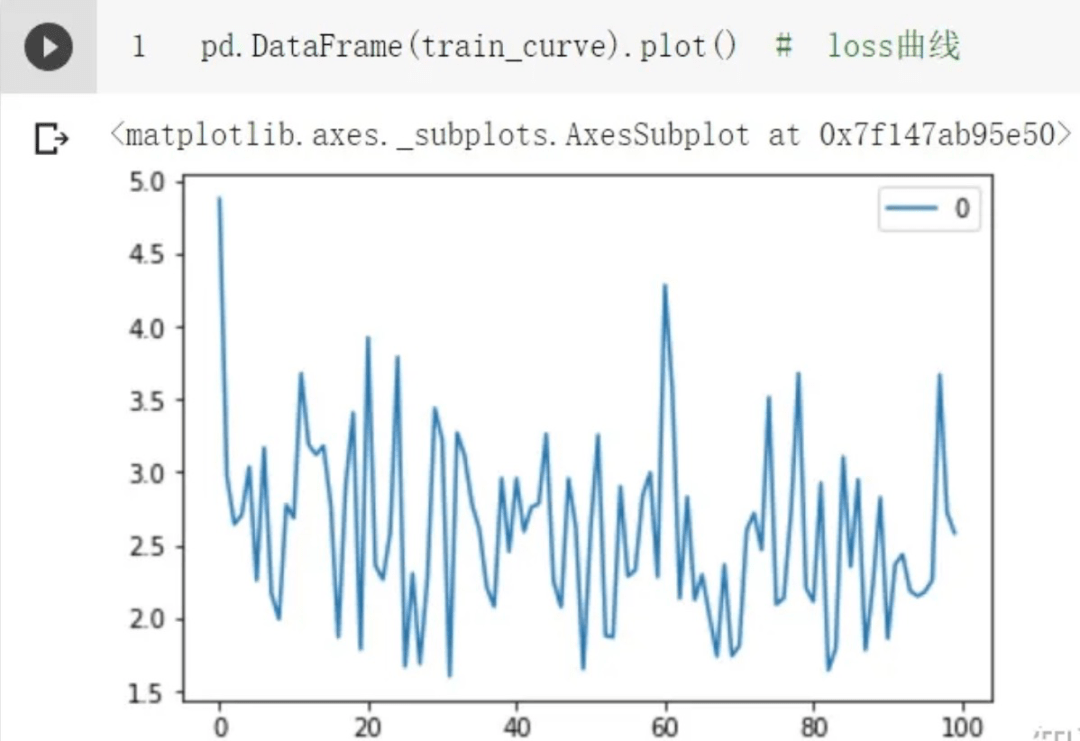Relevant code links are as follows:

BERT text classification jupyter version

BERT text classification pytorch version

## 2.1 TextCNN

Before the advent of Bert, TextCNN occupied a pivotal position in text classification models. This is because the CNN network can effectively capture the n-gram information in the text sequence, and the classification task is essentially to capture the n-gram permutation and combination features. Whether it is keywords, content, or the upper-level semantics of the sentence, they all exist in the form ofn-gramfeatures in the sentence.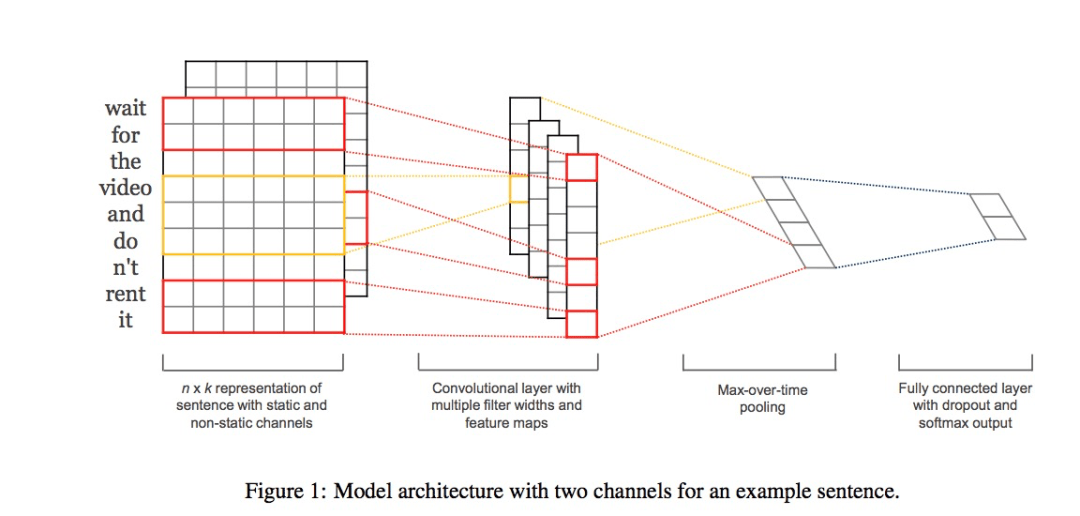TextCNN model structure

## 2.2 Magical Reform Ideas

After conducting experiments on Bert and TextCNN, the author was surprised to find that Bert can often better classify some sentences with obscure expressions, and TextCNN is often more sensitive to keywords. So the author modified the model and merged the ideas of Bert and TextCNN.

Bert-Base has 12 encoder layers except the first input layer. The first token (CLS) vector of each encoder layer can be used as a sentence vector. We can understand it abstractly as:

• The shallower the encode layer, the better the sentence vector can represent low-level semantic information;

• The deeper it is, the higher level semantic information it represents.

Our goal is to obtain both word-related features and semantic features. The specific approach of the model is to use the CLS vectors from layer 1 to layer 12 as input to the CNN and then classify them.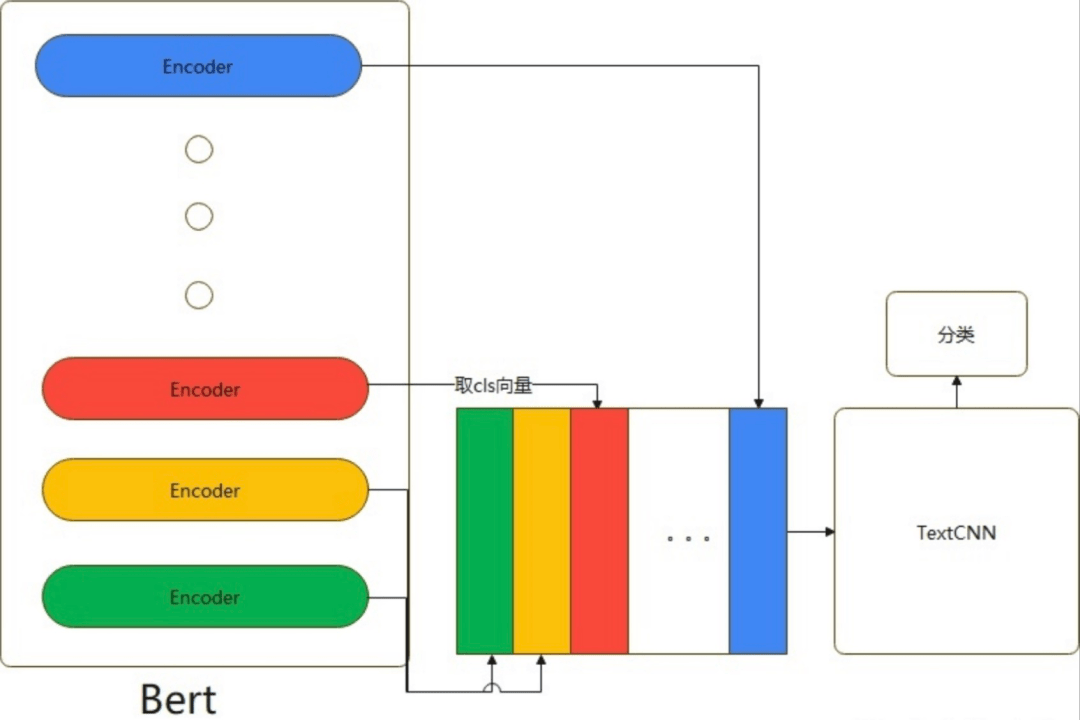Fusion BERT-Blend-CNN

Without further ado, let’s just look at the code!

## 2.3 pytorch code implementation

```# -*- coding:utf-8 -*-
#bert combines textcnn ideas with Bert + Blend-CNN
# model: Bert + Blend-CNN

import os
import numpy as np
import pandas as pd
import torch
import torch.nn as nn
import torch.utils.data as Data
import torch.nn.functional as F
import torch.optim as optim
import transformers
from transformers import AutoModel, AutoTokenizer
import matplotlib.pyplot as plt

train_curve = []
device = torch.device('cuda' if torch.cuda.is_available() else 'cpu')

# # Define some parameters and choose the most basic BERT Chinese model for the model.
batch_size = 2
epoches = 100
model = "bert-base-chinese"
hidden_size = 768
n_class = 2
maxlen = 8

encode_layer=12
filter_sizes = [2, 2, 2]
num_filters = 3

#data, construct some training data
sentences = ["I like playing basketball", "This camera is very nice", "I had a lot of fun today", "I don't like you", "Too bad", " It's such a sad thing"]
labels = [1, 1, 1, 0, 0, 0] # 1 positive, 0 negative.

class MyDataset(Data.Dataset):
def __init__(self, sentences, labels=None, with_labels=True,):
self.tokenizer = AutoTokenizer.from_pretrained(model)
self.with_labels = with_labels
self.sentences = sentences
self.labels = labels
def __len__(self):
return len(sentences)

def __getitem__(self, index):
# Selecting sentence1 and sentence2 at the specified index in the data frame
sent = self.sentences[index]

# Tokenize the pair of sentences to get token ids, attention masks and token type ids
encoded_pair = self.tokenizer(sent,
truncation=True, # Truncate to max_length
max_length=maxlen,

token_ids = encoded_pair['input_ids'].squeeze(0) # tensor of token ids
attn_masks = encoded_pair['attention_mask'].squeeze(0) # binary tensor with "0" for padded values and "1" for the other values
token_type_ids = encoded_pair['token_type_ids'].squeeze(0) # binary tensor with "0" for the 1st sentence tokens & amp; "1" for the 2nd sentence tokens

if self.with_labels: # True if the dataset has labels
label = self.labels[index]
else:

train = Data.DataLoader(dataset=MyDataset(sentences, labels), batch_size=batch_size, shuffle=True, num_workers=1)

class TextCNN(nn.Module):
def __init__(self):
super(TextCNN, self).__init__()
self.num_filter_total = num_filters * len(filter_sizes)
self.Weight = nn.Linear(self.num_filter_total, n_class, bias=False)
self.bias = nn.Parameter(torch.ones([n_class]))
self.filter_list = nn.ModuleList([
nn.Conv2d(1, num_filters, kernel_size=(size, hidden_size)) for size in filter_sizes
])

def forward(self, x):
# x: [bs, seq, hidden]
x = x.unsqueeze(1) # [bs, channel=1, seq, hidden]

pooled_outputs = []
for i, conv in enumerate(self.filter_list):
h = F.relu(conv(x)) # [bs, channel=1, seq-kernel_size + 1, 1]
mp = nn.MaxPool2d(
kernel_size = (encode_layer-filter_sizes[i] + 1, 1)
)
# mp: [bs, channel=3, w, h]
pooled = mp(h).permute(0, 3, 2, 1) # [bs, h=1, w=1, channel=3]
pooled_outputs.append(pooled)

h_pool = torch.cat(pooled_outputs, len(filter_sizes)) # [bs, h=1, w=1, channel=3 * 3]
h_pool_flat = torch.reshape(h_pool, [-1, self.num_filter_total])

output = self.Weight(h_pool_flat) + self.bias # [bs, n_class]

return output

# model
class Bert_Blend_CNN(nn.Module):
def __init__(self):
super(Bert_Blend_CNN, self).__init__()
self.bert = AutoModel.from_pretrained(model, output_hidden_states=True, return_dict=True)
self.linear = nn.Linear(hidden_size, n_class)
self.textcnn = TextCNN()

def forward(self, X):
input_ids, attention_mask, token_type_ids = X, X, X
# Get the vector encoded by each layer
# outputs.pooler_output: [bs, hidden_size]
hidden_states = outputs.hidden_states # 13*[bs, seq_len, hidden] The first layer is the embedding layer and is not required
cls_embeddings = hidden_states[:, 0, :].unsqueeze(1) # [bs, 1, hidden]
# Extract the first token (cls vector) of each layer and put them together as the input of textcnn
for i in range(2, 13):
cls_embeddings = torch.cat((cls_embeddings, hidden_states[i][:, 0, :].unsqueeze(1)), dim=1)
# cls_embeddings: [bs, encode_layer=12, hidden]
logits = self.textcnn(cls_embeddings)
return logits

bert_blend_cnn = Bert_Blend_CNN().to(device)

loss_fn = nn.CrossEntropyLoss()

#train
sum_loss = 0
total_step = len(train)
for epoch in range(epoches):
for i, batch in enumerate(train):
batch = tuple(p.to(device) for p in batch)
pred = bert_blend_cnn([batch, batch, batch])
loss = loss_fn(pred, batch)
sum_loss + = loss.item()

loss.backward()
optimizer.step()
if epoch % 10 == 0:
print('[{}|{}] step:{}/{} loss:{:.4f}'.format(epoch + 1, epoches, i + 1, total_step, loss.item()))
train_curve.append(sum_loss)
sum_loss = 0

# test
bert_blend_cnn.eval()
test_text = ['I don't like playing basketball']
test = MyDataset(test_text, labels=None, with_labels=False)
x = test.__getitem__(0)
x = tuple(p.unsqueeze(0).to(device) for p in x)
pred = bert_blend_cnn([x, x, x])
pred = pred.data.max(dim=1, keepdim=True)
if pred == 0:
print('negative')
else:
print('positive')

pd.DataFrame(train_curve).plot() # loss curve

```

## 2.4 Test results and code links

Test single sample result: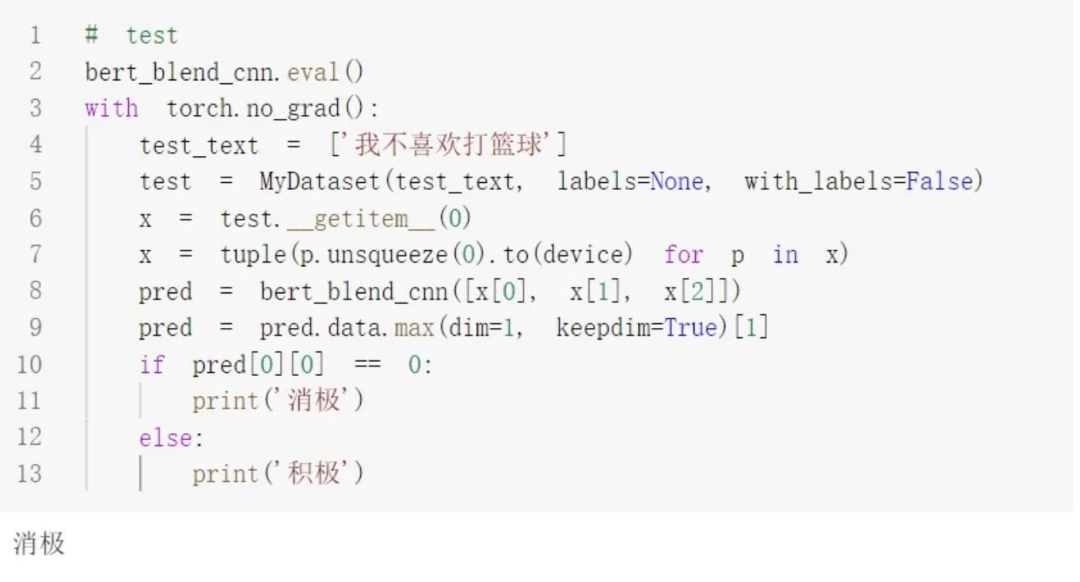loss curve: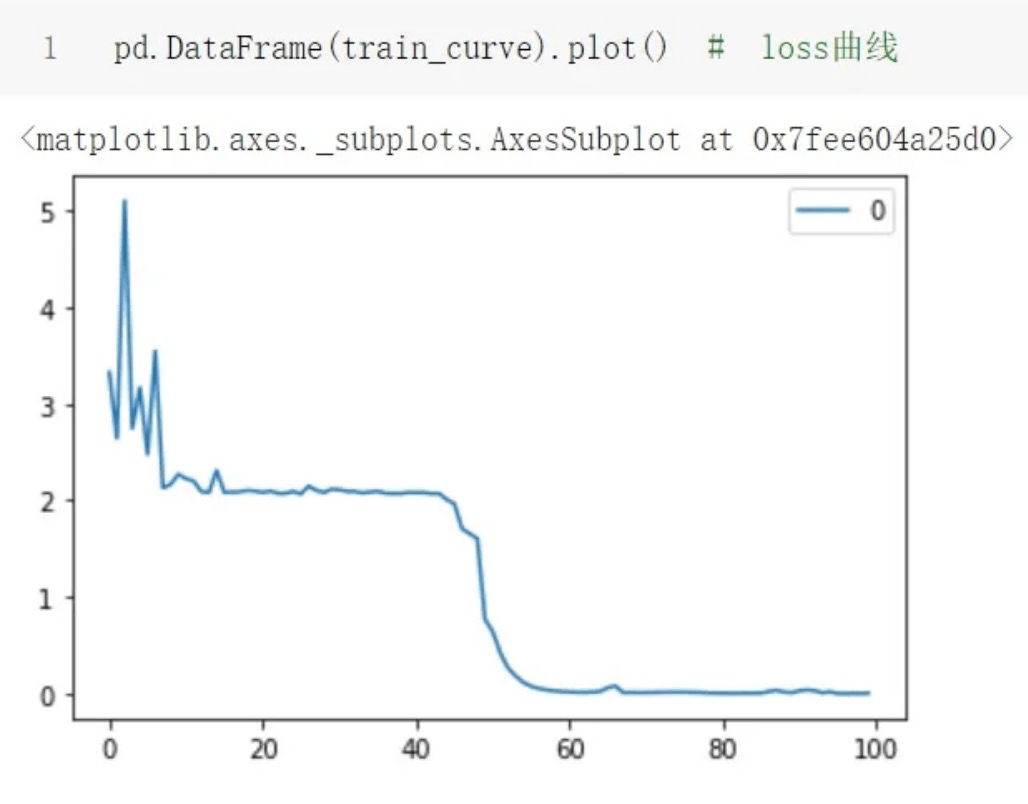BERT-Blend-CNNjupyter version

BERT-Blend-CNNpytorch version

### Reference materials

《The Illustrated Transformer》: https://jalammar.github.io/illustrated-transformer/

 BERT text classification jupyter version: https://github.com/PouringRain/blog_code/blob/main/nlp/bert_classify.ipynb

BERT text classification pytorch version: https://github.com/PouringRain/blog_code/blob/main/nlp/bert_classify.py

BERT-Blend-CNNjupyter version: https://github.com/PouringRain/blog_code/blob/main/nlp/Bert_Blend_CNN.ipynb

BERT-Blend-CNNpytorch version: https://github.com/PouringRain/blog_code/blob/main/nlp/bert_blend_cnn.py